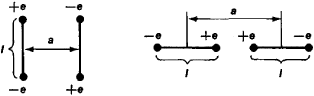Also found in: Dictionary.

[′kwä·drə‚pōl]
(electromagnetism)
A distribution of charge or magnetization which produces an electric or magnetic field equivalent to that produced by two electric or magnetic dipoles whose dipole moments have the same magnitude but point in opposite directions, and which are separated from each other by a small distance.
(mathematics)
A mass distribution that has unequal components of the moment-of-inertia tensor along the three principal directions.
McGraw-Hill Dictionary of Scientific & Technical Terms, 6E, Copyright © 2003 by The McGraw-Hill Companies, Inc.
The following article is from The Great Soviet Encyclopedia (1979). It might be outdated or ideologically biased.

a system of charged particles whose total electric charge and electric dipole moment equal zero.Figure 1. Examples of relative location of dipoles in a quadrupole

A quadrupole can be considered a set of two identical dipoles with the dipole moments equal in magnitude but opposite in direction and separated by a certain distance. At great distances R from the quadrupole, the field intensity of the quadrupole E decreases in inverse proportion to R to the fourth power (E∼ 1/R4). The relationship between E and the charges and their location is described in the general case by a set of five independent values that together constitute the quadrupole moment of the system. The quadrupole moment also determines the energy of a quadrupole in an external electric field. In the case described by Figure 1 the absolute value of the quadrupole moment is equal to 2ela, where e is the charge, 1 is the dimension of the dipoles, and a is the distance between the centers of the dipoles. A quadrupole is a second-order multipole.

### REFERENCE

Landau, L. D., and E. M. Lifshits. Teoriia polia, 5th ed. Moscow, 1967, § 41.

G. IA. MIAKISHEV